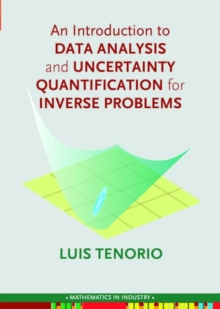Supporting your high street Find out how »
• My Account# An Introduction to Data Analysis and Uncertainty Quantification for Inverse Problems Paperback / softback

## Part of the Mathematics in Industry series

#### Description

Inverse problems are found in many applications, such as medical imaging, engineering, astronomy, and geophysics, among others.

To solve an inverse problem is to recover an object from noisy, usually indirect observations.

Solutions to inverse problems are subject to many potential sources of error introduced by approximate mathematical models, regularization methods, numerical approximations for efficient computations, noisy data, and limitations in the number of observations; thus it is important to include an assessment of the uncertainties as part of the solution.

Such assessment is interdisciplinary by nature, as it requires, in addition to knowledge of the particular application, methods from applied mathematics, probability, and statistics.This book bridges applied mathematics and statistics by providing a basic introduction to probability and statistics for uncertainty quantification in the context of inverse problems, as well as an introduction to statistical regularization of inverse problems.

The author covers basic statistical inference, introduces the framework of ill-posed inverse problems, and explains statistical questions that arise in their applications.An Introduction to Data Analysis and Uncertainty Quantification for Inverse Problems includes:many examples that explain techniques which are useful to address general problems arising in uncertainty quantificationBayesian and non-Bayesian statistical methods and discussions of their complementary roles, andanalysis of a real data set to illustrate the methodology covered throughout the book.

#### Information

• Format: Paperback / softback
• Pages: 269 pages
• Publisher: Society for Industrial & Applied Mathematics,U.S.
• Publication Date:
• Category: Differential calculus & equations
• ISBN: 9781611974911

£66.50

£66.35

on all orders

###### Pick up orders

from local bookshops

£159.99

£159.50

£135.58

£69.99

£69.65

£70.00

£59.50